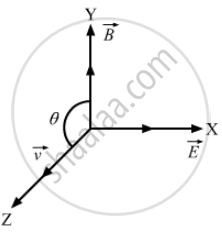Department of Pre-University Education, KarnatakaPUC Karnataka Science Class 11

# The Force on a Charged Particle Due to Electric and Magnetic Fields is Given by → F = Q → E + Q → ν × → B → E is Along the X-axis and → B Along the Y-axis. in What Direction - Physics

Sum

The force on a charged particle due to electric and magnetic fields is given by $\vec{F} = q \vec{E} + q \vec{\nu} \times \vec{B}$.
Suppose $\vec{E}$  is along the X-axis and $\vec{B}$  along the Y-axis. In what direction and with what minimum speed ν should a positively charged particle be sent so that the net force on it is zero?

#### Solution

According to the problem, the net electric and magnetic forces on the particle should be zero.i.e.,
$\vec{F} = q \vec{E} + q\left( \vec{\nu} \times \vec{B} \right) = 0$
$\Rightarrow E = - \left( \vec{\nu} \times \vec{B} \right)$
So, the direction of $\vec{\nu} \times \vec{B}$  should be opposite to the direction of $\vec{E}$ Hence, $\vec{\nu}$ should be along the positive z-direction.
Again, E = νB sin θ
$\Rightarrow \nu = \frac{E}{B} \sin \theta$
For ν to be minimum, $\theta = 90 \text{ and }, thus, \nu_\min = \frac{E}{B}$
So, the particle must be projected at a minimum speed of $\frac{E}{B}$ along the z-axis.

Concept: Physics Related to Technology and Society
Is there an error in this question or solution?

#### APPEARS IN

HC Verma Class 11, Class 12 Concepts of Physics Vol. 1
Chapter 2 Physics and Mathematics
Exercise | Q 18 | Page 29

Share